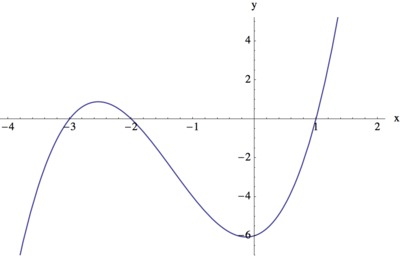Graphing from Factors III

Alignments to Content Standards: A-APR.B.3 A-APR.B.2

Mike is trying to sketch a graph of the polynomial $$f(x)=x^3+4x^2+x-6.$$ He notices that the coefficients of $f(x)$ add up to zero $(1 + 4 + 1 -6 = 0)$ and says

This means that 1 is a root of $f(x)$, and I can use this to help factor $f(x)$ and produce the graph.
1. Is Mike right that 1 is a root of $f(x)$? Explain his reasoning.
2. Find all roots of $f(x)$.
3. Find all inputs $x$ for which $f(x)\lt 0$.
4. Use the information you have gathered to sketch a rough graph of $f$.

IM Commentary

The task has students use the remainder theorem to deduce a linear factor of a cubic polynomial, and then to completely factor the polynomial. Students will need some procedure (e.g., synthetic or long division, or guess-and-check the coefficients) for determining the quadratic factor. Having the factored form permits students to deduce much about the structure of the graph. In addition to having learned the $x$-intercepts, writing the polynomial as $$x^3+4x^2+x-6=(x-1)(x+2)(x+3)$$ also reveals, for example, where the function is negative: indeed, to give a negative output, exactly one of the three factors (or all three factors) has to be negative, giving $x\lt -3$ or $-2\lt x\lt 1$.

The task can be the basis for a classroom activity and discussion. In this task students engage in SMP 7 - Look for and make use of structure. Students use the structure of the polynomial in factored form to make deductions about the structure of the graph.

Solution

1. Mike is right that 1 is a root of $f(x)$ and we verify this by evaluating $f(1)$, we also see why his reasoning works:

\begin{align} f(1) &= 1^3 + 4 \times 1^2 + 1 \times 1 - 6 \\ &= 1^3 + 4 + 1 - 6 \\ &= 0. \end{align}
The key here is that when we substitute 1 into $f(x)$, all of the powers of $x$ are 1, and so the value of $f(1)$ is simply the sum of the coefficients of $f(x)$. Since the sum of the coefficients of $f(x)$ is 0 this means that $f(1) = 0$ and so 1 is a root of $f(x)$.

2. By the remainder theorem, since $1$ is a root of $f(x)$, the linear term $x-1$ is a factor of $f$: that is, when we divide $f(x)$ by $x-1$ we know that there will be zero remainder. We find (e.g., by long division, or synthetic division, among other choices) $$f(x) = (x-1)(x^2 + 5x + 6).$$ Since $x^2 + 5x + 6$ factors as $(x+2)(x+3)$, we conclude $$f(x) = (x-1)(x+2)(x+3).$$

3. We can use the factored form of $f(x)$ to deduce where $f$ is positive or negative. Since a product of three real numbers is negative when all three are negative, or when exactly one is negative, $f(x)$ gives a negative output when one of two things happens:

• All of $x-1$, $x+2$, and $x+3$ are negative. Since $x+3$ is the largest of the three, this happens when $x+3\lt 0$, which is when $x\lt -3$.
• Exactly one of the three linear terms is negative. Since $x-1\lt x+2\lt x+3$, this could only be true if $x-1\lt 0$ but $x+2\gt 0$, that is, $-2\lt x\lt 1$.
So $f(x)$ is negative if and only if $-2\lt x\lt 1$ or $x\lt -3$.

4. Since $f(x)$ is a cubic polynomial with positive coefficient for $x^3$ this means that it will take large positive values when $x$ is large and positive and large negative values when $x$ is large and negative. This, together with the knowledge of where $f(x)$ takes the value 0, and where $f(x)$ is negative, allows us to draw the following sketch for the graph of $y=f(x)$: